An official website of the United States governmentOfficial websites use .gov
A .gov website belongs to an official government organization in the United States.Secure .gov websites use HTTPS
A lock ( ) or https:// means you've safely connected to the .gov website. Share sensitive information only on official, secure websites.

# NIST Guide to the SI, Chapter 10: More on Printing and Using Symbols and Numbers in Scientific and Technical Documents

## Share

By following the guidance given in this chapter, NIST authors can prepare manuscripts that are consistent with accepted typesetting practice.

10.1 Kinds of symbols

Letter symbols are of three principal kinds: (a) symbols for quantities, (b) symbols for units, and (c) symbols for descriptive terms. Quantity symbols, which are always printed in italic (that is, sloping) type, are, with few exceptions, single letters of the Latin or Greek alphabets that may have subscripts or superscripts or other identifying signs. Symbols for units, in particular those for acceptable units, have been discussed in detail in earlier portions of this Guide. Symbols for descriptive terms include the symbols for the chemical elements, certain mathematical symbols, and modifying superscripts and subscripts on quantity symbols.

10.1.1 Standardized quantity symbols

The use of words, acronyms, or other ad hoc groups of letters as quantity symbols should be avoided by NIST authors. For example, use the quantity symbol Zm for mechanical impedance, not MI. In fact, there are nationally and internationally accepted symbols for literally hundreds of quantities used in the physical sciences and technology. Many of these are given in Refs.  and , and it is likely that symbols for the quantities used in most NIST publications can be found in these international standards or can readily be adapted from the symbols and principles given in these standards. Because of their international acceptance, NIST authors are urged to use the symbols of Refs.  and  to the fullest extent possible.5

Examples:

 Ω (solid angle) Zm (mechanical impedance) LP (level of a power quantity) Δr (relative mass excess) p (pressure) σtot (total cross section) κT (isothermal compressibility) Eu (Euler number) E (electric field strength) TN (Néel temperature)

10.1.2 Standardized mathematical signs and symbols

As is the case for quantity symbols, most of the mathematical signs and symbols used in the physical sciences and technology are standardized. They may be found in Ref. [4: ISO 31-11] and should be used by NIST authors to the fullest possible extent.5

Examples:

 ^ (conjunction sign, p ^ q means p and q) ≠ (a ≠ b, a is not equal to b)( ab, a is by definition equal to b) ≈ (a ≈ b, a is approximately equal to b) ~ (a ~ b, a is proportionally equal to b) arcsin x (arc sine of x) logax (logarithm to the base a of x) lb x (lb x = log2x) ln x (ln x = logex) lg x (lg x = log10x)

10.2 Typefaces for symbols

Most word processing systems now in use at NIST are capable of producing lightface (that is, regular) or boldface letters of the Latin or Greek alphabets in both roman (upright) and italic (sloping) types. The understandability of NIST typed and typeset scientific and technical publications is facilitated if symbols are in the correct typeface. The typeface in which a symbol appears helps to define what the symbol represents. For example, irrespective of the typeface used in the surrounding text, "A" would be typed or typeset in:

— italic type for the scalar quantity area: A;
— roman type for the unit ampere: A;
— italic boldface for the vector quantity vector potential: A.

More specifically, the three major categories of symbols found in scientific and technical publications should be typed or typeset in either italic or roman type, as follows:

—symbols for quantities and variables: italic;
—symbols for units: roman;
—symbols for descriptive terms: roman.

These rules imply that a subscript or superscript on a quantity symbol is in roman type if it is descriptive (for example, if it is a number or represents the name of a person or a particle); but it is in italic type if it represents a quantity, or is a variable such as x in Ex or an index such as i in ∑ixi that represents a number (see Secs. 10.2.1, 10.2.3, and 10.2.4). An index that represents a number is also called a "running number" [4: ISO 31-0]

Notes:

1. The above rules also imply, for example, that μ, the symbol for the SI prefix micro (10-6), that Ω, the symbol for the SI derived unit ohm, and that F, the symbol for the SI derived unit farad, are in roman type; but they are in italic type if they represent quantities (μ, Ω, and F are the recommended symbols for the quantities magnetic moment of a particle, solid angle, and force, respectively).

2. The typeface for numbers is discussed in Sec. 10.5.1. The following four sections give examples of the proper typefaces for these three major categories.

10.2.1 Quantities and variables — italic

Symbols for quantities are italic, as are symbols for functions in general, for example, f (x):

 t = 3s time, s second T = 22 K T temperature, K kelvin r = 11 cm r radius, cm centimeter λ = 633 nm λ wavelength, nm nanometer

Constants are usually physical quantities and thus their symbols are italic; however, in general, symbols used as subscripts and superscripts are roman if descriptive (see Sec. 10.2.3):

 NA Avogadro constant, A Avogadro R molar gas constant θD Debye temperature, D Debye Z atomic number e elementary charge me m mass, e electron

Running numbers and symbols for variables in mathematical nist-equations are italic, as are symbols for parameters such as a and b that may be considered constant in a given context: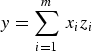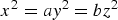Symbols for vectors are boldface italic, symbols for tensors are sans-serif bold italic, and symbols for matrices are italic:

A·B = C (vectors)           T (tensors)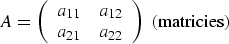Symbols used as subscripts and superscripts are italic if they represent quantities or variables:

Cpp pressure qmm mass      σΩ Ω solid angle ω zz   z coordinate

10.2.2 Units— roman

The symbols for units and SI prefixes are roman:

 m meter g gram L liter cm centimeter μg centimeter mL milliliter

10.2.3 Descriptive terms — roman

Symbols representing purely descriptive terms (for example, the chemical elements) are roman, as are symbols representing mathematical constants that never change (for example, π) and symbols representing explicitly defined functions or well defined operators (for example, Γ(x) or div): Chemical elements:

Ar      argon      B      boron      C      carbon

Mathematical constants, functions, and operators:

 e base of natural logarithms Σxi Σ sum of exp x exp exponential of logax loga logarithm to the base a of dx/dt d 1st derivative of sin x sin sine of

Symbols used as subscripts and superscripts are roman if descriptive:

 ε0(ir) ir irrational Ek k kinetic V1m m molar, l liquid phase μB B Bohr

10.2.4 Sample nist-equations showing correct type

 $$F = \frac{q_1 q_2}{4\pi \varepsilon_0 r^2}$$ F = ma pV = nRT $$\varphi_{\rm B} = x_{\rm B} V_{\rm m,B}^* /\sum x_{\rm A} V_{\rm m,A}^*$$ Ea = RT2 d(ln k)/dT $$c_1 = \lambda^{-5} / [\exp(c_2/\lambda T) - 1]$$ E = mc2 $$\tilde{p}_{\rm B} = \lambda_{\rm B} \lim_{p\rightarrow0} (x_{\rm B} p/\lambda_{\rm B})$$ $$\frac{F}{Q} = - {\bf grad} \, V$$

10.3 Greek alphabet in roman and italic type

Table 13 shows the proper form, in both roman and italic type, of the upper-case and lower-case letters of the Greek alphabet.

### Table 13. Greek alphabet in roman and italic type

Some browsers may not display this table as author intended. If in doubt please use the pdf version.

NameCapital
Roman
Lower Case
Roman
Capital
Italic
Lower Case
Italic
alphaAαAα
betaBβBβ
gammaΓγΓγ
deltaΔδΔδ
epsilonEεEε,ε
zetaZζZζ
etaHηHη
thetaΘ, Ө(a)θ,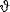(b)
Θ, Ө(a)θ,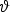(b)
iotaIιIι
kappaKκ, κ(b)Kκ, κ(b)
lambdaΛλΛλ
muMµMµ
nuNνNν
xiΞξΞξ
omicronOoOo
piΠπ,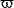Ππ,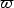rhoPρ, ρ(b)Pρ, ρ(b)
sigmaΣσΣσ
tauTτTτ
upsilonΥυΥυ
phiΦφ,φΦφ,φ
chiXχXχ
psiΨψΨψ
omegaΩωΩω

(a) ISO (see Ref. [4: ISO 31-0]) gives only the first of these two letters.
(b) ISO (see Ref. [4: ISO 31-0]) gives these two letters in the reverse order.

10.4 Symbols for the elements

The following two sections give the rules and style conventions for the symbols for the elements.

10.4.1 Typeface and punctuation for element symbols

Symbols for the elements are normally printed in roman type without regard to the type used in the surrounding text (see Sec. 10.2.3). They are not followed by a period unless at the end of a sentence.

10.4.2 Subscripts and superscripts on element symbols

The nucleon number (mass number) of a nuclide is indicated in the left superscript position: 28Si.

The number of atoms in a molecule of a particular nuclide is shown in the right subscript

position: 1H2. The proton number (atomic number) is indicated in the left subscript position: 29Cu.

The state of ionization or excitation is indicated in the right superscript position, some examples of which are as follows:

State of ionization: Ba++

Co(NO2)6---    or Co(NO2 )36-    or    [Co(NO2)6]3-

Electronic excited state: Ne*, CO*

Nuclear excited state: 15N* or 15Nm

10.5 Printing numbers

The following three sections give rules and style conventions related to the printing of numbers.

10.5.1 Typeface for numbers

Arabic numerals expressing the numerical values of quantities (see Sec. 7.6) are generally printed in lightface (that is, regular) roman type irrespective of the type used for the surrounding text. Arabic numerals other than numerical values of quantities may be printed in lightface or bold italics, or in bold roman type, but lightface roman type is usually preferred.

10.5.2 Decimal sign or marker

The recommended decimal sign or marker for use in the United States is the dot on the line [3, 6]. For numbers less than one, a zero is written before the decimal marker. For example, 0.25 s is the correct form, not .25 s.

10.5.3 Grouping digits

Because the comma is widely used as the decimal marker outside the United States, it should not be used to separate digits into groups of three. Instead, digits should be separated into groups of three, counting from the decimal marker towards the left and right, by the use of a thin, fixed space. However, this practice is not usually followed for numbers having only four digits on either side of the decimal marker except when uniformity in a table is desired.

Examples:

 76 483 522 but not: 76,483,522 43 279.168 29 but not: 43,279.168 29 8012 or 8 012 but not: 8,012 0.491 722 3 is highly preferred to: 0.4917223 0.5947 or 0.594 7 but not: 0.59 47 8012.5947 or 8 012.594 7 but not: 8 012.5947 or 8012.594 7

Note: The practice of using a space to group digits is not usually followed in certain specialized applications, such as engineering drawings and financial statements.

10.5.4 Multiplying numbers

When the dot is used as the decimal marker as in the United States, the preferred sign for the multiplication of numbers or values of quantities is a cross (that is, multiplication sign) (×), not a half-high (that is, centered) dot (·).

Examples:

 25 × 60.5 but not: 25·60.5 53 m/s × 10.2 s but not: 53 m/s·10.2 s 15 × 72 kg but not: 15·72 kg

Notes:

1. When the comma is used as the decimal marker, the preferred sign for the multiplication of numbers is the half-high dot. However, even when the comma is so used, this Guide prefers the cross for the multiplication of values of quantities.

2. The multiplication of quantity symbols (or numbers in parentheses or values of quantities in parentheses) may be indicated in one of the following ways: ab, a b, a·b, a × b.

Created January 28, 2016, Updated September 7, 2016